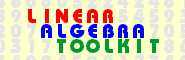© 2000−2019  P. Bogacki Solving a system of linear equations v. 1.25

 PROBLEM

Solve the following system of 3 linear equations in 3 unknowns:

 1 x1 -3 x2 +1 x3 = 1 2 x1 -1 x2 -2 x3 = -1 2 x1 -6 x2 +2 x3 = 2

 SOLUTION

 Step 1: Transform the augmented matrix to the reduced row echelon form  (Show details)

 1 -3 1 1 2 -1 -2 -1 2 -6 2 2

can be transformed by a sequence of elementary row operations to the matrix

 1 0 -7  5 -4  5 0 1 -4  5 -3  5 0 0 0 0

 Step 2: Interpret the reduced row echelon form

The reduced row echelon form of the augmented matrix is

 1 0 -7  5 -4  5 0 1 -4  5 -3  5 0 0 0 0

which corresponds to the system

 1 x1 +(-7/5) x3 = (-4/5) 1 x2 +(-4/5) x3 = (-3/5) 0 = 0

No equation of this system has a form zero = nonzero; Therefore, the system is consistent.

The leading entries in the matrix have been highlighted in yellow.

A leading entry on the (i,j) position indicates that the j-th unknown will be determined using the i-th equation.

Those columns in the coefficient part of the matrix that do not contain leading entries, correspond to unknowns that will be arbitrary. The system has infinitely many solutions:

 x1 = +(7/5) x3 +(-4/5) x2 = +(4/5) x3 +(-3/5) x3 = arbitrary

This concludes the solution of the problem. Do you want to
• solve another problem of the same type, or
• go to the main Toolkit page?